# SAT II Physics : Velocity, Frequency, and Wavelength

## Example Questions

### Example Question #1 : Frequency And Period

What is the wavelength of a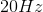wave if its velocity is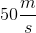?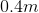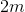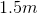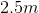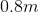Explanation:

The relationship between wavelength and velocity is given by the equation;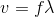The question gives us the velocity of the wave and its frequency. Using these values, we can solve for the wavelength.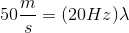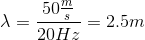### Example Question #1 : Waves

What best describes how a wave is affected when it travels from one medium to another medium?

Neither speed not frequency change

The wavelength does not change

Both speed and frequency change

The wave speed changed, but the frequency remains the same

The frequency changes, but the speed remains the same